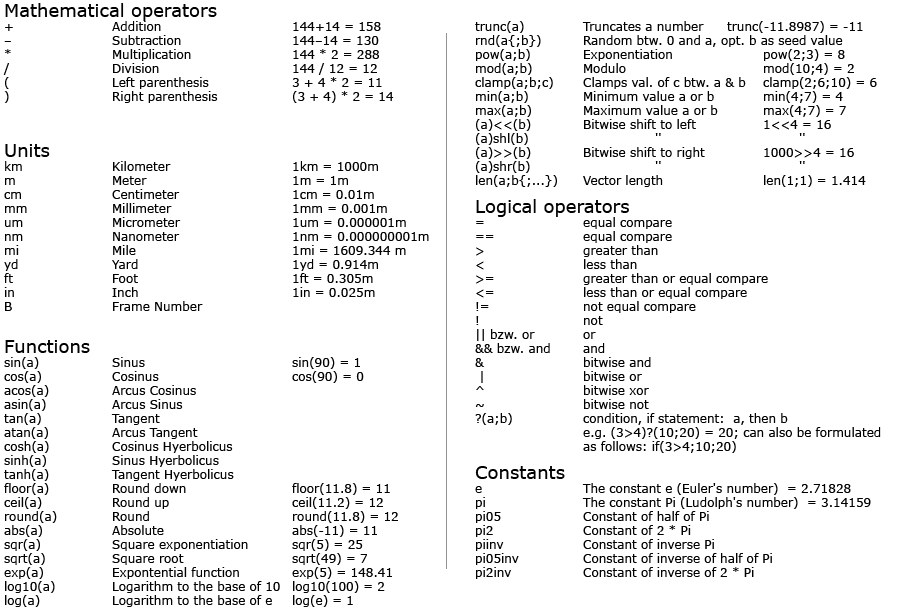Formula

# General

You can type in a formula for the Formula spline primitive and the Formula deformer. In fact, you can type in a formula wherever Cinema 4D accepts a value, e.g., in the Attribute Manager. This appendix lists all the units, operators, functions and constants that you may use in your formulae. You may enter values using any of the units listed below (examples in brackets) - regardless of the basic units defined in the preferences (Cinema 4D will convert the units for you).

Arguments such as mod(a;b) must be separated by a semi-colon or by squared brackets [] (then without curved brackets)

rnd(100) is the same as rnd and rnd(100;234) is the same as rnd().Curly brackets (e.g., rnd(a{;b})) represent optional, i.e., must not necessarily be used, values.

Note the special variables in association with multiple selections in the following paragraph.

## Expanded Formula Entry by Multiple Selections

You can also make relative adjustments to multiple selected objects. For example, suppose a sphere is positioned at (100,0,0) and a cube at (300,0,0). Select both objects and in the Attribute Manager, on the Coord tab, enter x + 100 into the P.X box. Both objects will be moved 100 units along the X axis. You can also enter equations, such as sin(x)+sqr(x*324).

The following variables are available:

x: Represents the parameter’s original value

num: Represents the index value. The selected elements/objects will be numbered

tot: The total number of selected elements/objects

rnd(value): A random value between 0 and "value" will be ascertained

## Examples

• You have three spheres at a position (parameter P.X) of 100, 200 and 300 meters, respectively. If you select all three spheres simultaneously and enter x+200 in the P.X field the spheres would be placed at 300, 400 and 500 meters, respectively.
• 10 light sources: You want to increase the intensity of the light sources incrementally in steps of 20, beginning at 0. Select all light sources and enter num*20 in the Intensity field. Corresponding to the order in which they appear in the Object Manager, the first light will have an intensity of 0, the second light an intensity of 20, the third an intensity of 40, etc.
• You want to randomly disperse the intensity of ten light sources over a total value of 200: Select all light sources and enter x+rnd(200) in the Intensity field. If all light sources previously had an intensity of 0, each should now have a random intensity within a total value of 200.
• You want to set the Fillet Radius for twenty eight cubes to values ranging from 0 to 100 with equal intervals: Select all cubes and enter 100*(num/(tot-1)) into the Fillet Radius field.Even enlargement of cube fillet radii.
Tip:If you want to define color values using this method, right-click on the Color parameter and select Show Subchannels from the context menu that appears. This method works with these Subchannels.

# Units

A custom value can be entered, independently of any preset values.

Tip:If you change the basic units in the preferences, e.g., from meters to millimeters, only the measurement units are changed, not existing numerical values. For example, if an object has a width of 10 meters, but you then change the basic units to millimeters, the object will then have a width of 10 millimeters. If you wish to scale the objects to reflect the change in units, group all the objects and scale the group using the Coordinate Manager.

# Functions

Tip:Function arguments must be bracketed. The number of open brackets must equal the number of close brackets. Functions may be nested: sin(sqr(exp(pi))).
Trigonometric function arguments will always be interpreted as degrees. Hence, the entry sin(2*pi) does not reflect the calculation of a sine of 360° but rather of approx. 6.283°.

# General

When typing in a formula for the spline or Formula time curve, the arguments of trigonometric functions are in radians. However, when entering values in parameter text boxes, trigonometric functions always use degrees.

The function parser has the most important arithmetic operators built in. You can combine operations freely, for example: 2km + exp(sin(4mm*pi)) / ((sin(14cm))^2 + (cos(14cm))^2).